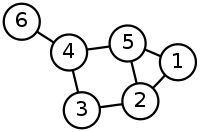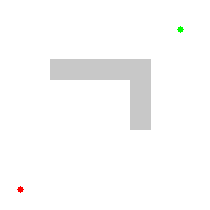# Using nodes

Introduction to how to use nodes.

Pathfinding is done on graphs of nodes. These nodes are connected to each other in various ways. In grids each tile is a node and they are connected to their 4 or 8 adjacent tiles. In navmesh/recast graphs each triangle is a node and the nodes are positioned in the centers of the triangles.Explaining how the whole search algorithm works is beyond the scope of this tutorial but here is a nice animation from Wikipedia:### See

Accessing graph data

### Finding Nodes close to Positions

Often you want to figure out which node is the closest one to a point in the world. Usually when just dealing with the built-in movement scripts you do not have to deal with nodes at all but as you dig deeper it becomes very useful. `// Find the closest node to this GameObject's positionGraphNode node = AstarPath.active.GetNearest(transform.position).node;if (node.Walkable) { // Yay, the node is walkable, we can place a tower here or something}` There are also cases where you want to get only certain nodes, such as "What is the closest *Walkable* node to this position?". This can be accomplished using the NNConstraint class. For this case the Default NNConstraint (it is called default, because it is used for path calls if nothing else is specified) `GraphNode node = AstarPath.active.GetNearest(transform.position, NNConstraint.Default).node;` You can also create custom constraints: `var constraint = NNConstraint.None;// Constrain the search to walkable nodes onlyconstraint.constrainWalkability = true;constraint.walkable = true;// Constrain the search to only nodes with tag 3 or tag 5// The 'tags' field is a bitmaskconstraint.constrainTags = true;constraint.tags = (1 << 3) | (1 << 5);var info = AstarPath.active.GetNearest(transform.position, constraint);var node = info.node;var closestPoint = info.position;` The search range of these constraints is not unlimited, but quite large. The maximum distance can be set in A* Inspector -> Settings -> Max Nearest Node Distance or AstarPath.maxNearestNodeDistance. If the closest node is further away than the 'Max Nearest Node Distance' then the GetNearest method will return a null node.

### See

The Pathfinding.NNConstraint class has a few more options that you can take a look at if you want.

#### Closest Point on Nodes

You may have noticed above that to get the node from the GetNearest call we had to access the node field. The GetNearest method returns an NNInfo struct which contains two fields. The first one is the node field which contains the best node (or null if no node could be found). The second one is the position field which contains the closest point on that node to the query point. On grid graphs, the nodes are thought of as squares so the position field will then contain the closest point inside that square to the query point. On navmesh based graphs (RecastGraph and NavMeshGraph) the position field will contain the closest point on the closest triangle. Point nodes do not have a surface so the position will just be set to the node's position.

`var info = AstarPath.active.GetNearest(transform.position);var node = info.node;var closestPoint = info.position;`

### Node connections

Each node stores which other nodes it is connected to. How this is represented depends on the graph. Grid graphs for example take advantage of its grid structure and can store all connections to its adjacent grid nodes in just a single byte! This is significantly more memory efficient compared to for example an array of references to other nodes as that would use at least 80 times more memory.

You can access all connections of a node using the GetConnections method. This method takes a delegate which will be called for each node it is connected to and abstracts away the underlaying representation.

`GraphNode node = ...;// Draw a line to all nodes that this node is connected tonode.GetConnections(otherNode => { Debug.DrawLine((Vector3)node.position, (Vector3)otherNode.position);});`

### See

You can also add or remove custom connections from nodes. For more information take a look at Writing Graph Generators.

### Node properties

There are a few methods on node instances that are of interest.

Firstly nodes have a position field which is the position of the node in world space. This position is stored as an Int3, not as a Vector3. You can however easily convert betwen Vector3s and Int3s. `GraphNode node = ...;Vector3 v3position = (Vector3)node.position;node.position = (Int3)v3position;`

You can also access various properties on the node such as if it is walkable, which tag it has and its penalty. `bool walkable = node.Walkable;uint tag = node.Tag;uint penalty = node.Penalty;`

### See

There are also some utility methods for finding points on nodes.

You can get a random point on the node's surface: `Vector3 randomPoint = node.RandomPointOnSurface();` and the surface area of the node (this will be zero for point nodes): `float surfaceArea = node.SurfaceArea();`

### TriangleMeshNode

Mesh nodes are used on navmesh and recast graphs.

### See

Graph Types

These have a few more utilities. You can get the closest point on just that node. Either in XZ space (i.e as if you would view it from above) or in 3D space. `MeshNode node = ...;Vector3 closestPoint = node.ClosestPointOnNode(somePoint);Vector3 closestPoint = node.ClosestPointOnNodeXZ(somePoint);` You can also check if a specific point is inside that node (when seen from above). `if (node.ContainsPoint(somePoint)) { // The point is inside the node}`

You can get the verties of the triangle that the node represents using `// IndividuallyInt3 v0 = node.GetVertex(0);Int3 v1 = node.GetVertex(1);Int3 v2 = node.GetVertex(2);`

`// Or combined (faster)Int3 v0, v1, v2;node.GetVertices(out v0, out v1, out v2);`

### Reachability

Often it is useful to determine if a character can reach a certain node. Keep in mind that when a path is calculated it will by default try to move to the closest node to the target that it can reach (up to a maximum distance of AstarPath.maxNearestNodeDistance as discussed earlier). So often you do not have to care about this.

The pathfinding system precalculates which nodes are reachable from which other nodes by calculating the connected components of the graphs. This is what is visible as the different colors in the scene view (when the Graph Coloring option is set to 'Areas'). Each node's Area field is set to the index of its connected component. If 2 nodes have the same area it means there is a valid path between them. You can also check this using the PathUtilities.IsPathPossible method. `var node1 = AstarPath.active.GetNearest(somePoint1);var node2 = AstarPath.active.GetNearest(somePoint2);if (PathUtilities.IsPathPossible(node1, node2)) { // There is a valid path between the nodes}` The process of calculating these areas or connected components is referred to as 'flood filling' in various parts of the documentation and code.

You can also supply a tag mask or a list of nodes to the IsPathPossible method.

### See

Pathfinding.PathUtilities

You can also find all nodes that can be reached from a given node. `List<GraphNode> reachableNodes = PathUtilities.GetReachableNodes(node);`

### See

There are also several ways of finding all nodes up to a specific distance from a node. For more info take a look at Wandering AI Tutorial.